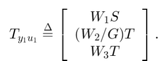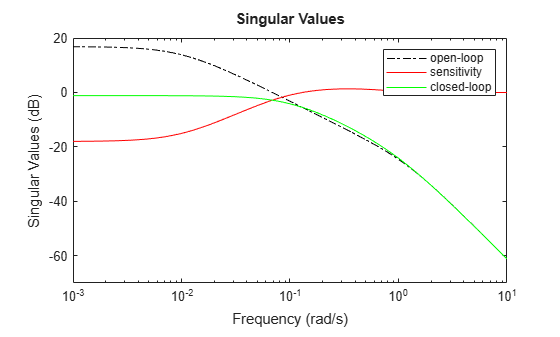# h2syn

Compute H2 optimal controller

## Syntax

``[K,CL,gamma] = h2syn(P,nmeas,ncont)``
``[K,CL,gamma] = h2syn(P,nmeas,ncont,opts)``
``[K,CL,gamma,info] = h2syn(___)``

## Description

example

````[K,CL,gamma] = h2syn(P,nmeas,ncont)` computes a stabilizing H2-optimal controller `K` for the plant `P`. The plant has a partitioned form$\left[\begin{array}{c}z\\ y\end{array}\right]=\left[\begin{array}{cc}{P}_{11}& {P}_{12}\\ {P}_{21}& {P}_{22}\end{array}\right]\left[\begin{array}{c}w\\ u\end{array}\right],$where: w represents the disturbance inputs.u represents the control inputs.z represents the error outputs to be kept small.y represents the measurement outputs provided to the controller.`nmeas` and `ncont` are the number of signals in y and u, respectively. y and u are the last outputs and inputs of `P`, respectively. `h2syn` returns a controller `K` that stabilizes `P` and has the same number of states. The closed-loop system `CL`` = lft(P,K)` achieves the performance level `gamma`, which is the H2 norm of `CL` (see `norm`).```
````[K,CL,gamma] = h2syn(P,nmeas,ncont,opts)` specifies additional computation options. To create `opts`, use `h2synOptions`. ```
````[K,CL,gamma,info] = h2syn(___)` returns a structure containing additional information about the H2 synthesis computation. You can use this argument with any of the previous syntaxes.```

## Examples

collapse all

Stabilize a 5-by-4 unstable plant with three states, two measurement signals, and one control signal.

In practice, `P` is an augmented plant that you have constructed by combining a model of the system to control with appropriate ${\mathit{H}}_{2}$ weighting functions. For this example, use the following model.

```A = [5 6 -6 6 0 5 -6 5 4]; B = [0 4 0 0 1 1 -2 -2 4 0 0 -3]; C = [-6 0 8 0 5 0 -2 1 -4 4 -6 -5 0 -15 7]; D = [0 0 0 0 0 0 0 1 0 0 0 0 0 0 3 6 8 0 -7 0]; P = ss(A,B,C,D);```

Confirm that `P` is unstable by examining its poles, some of which lie in the right half-plane.

`pole(P)`
```ans = 3×1 -8.5648 6.8612 10.7036 ```

Design the stabilizing controller. `h2syn` assumes that the `nmeas` measurement signals and the `ncont` control signals are the last outputs and last inputs of `P`, respectively.

```nmeas = 2; ncont = 1; [K,CL,gamma] = h2syn(P,nmeas,ncont);```

Examine the closed-loop system to confirm that the controller `K` stabilizes the plant.

`pole(CL)`
```ans = 6×1 complex -31.6236 + 0.0000i -12.6460 + 3.8045i -12.6460 - 3.8045i -9.6073 + 0.0000i -9.2393 + 0.0000i -8.6939 + 0.0000i ```

Shape the singular value plots of the sensitivity $S=\left(I+GK{\right)}^{-1}$ and complementary sensitivity $T=GK\left(I+GK{\right)}^{-1}$.

To do so, find a stabilizing controller `K` that minimizes the ${H}_{2}$ norm of:Assume the following plant and weights:

`$G\left(s\right)=\frac{s-1}{s-2},{W}_{1}=\frac{0.1}{100s+1},{W}_{2}=0.1,{W}_{3}=0.$`

Using those values, construct the augmented plant `P`, as illustrated in the `mixsyn` reference page.

```s = zpk('s'); G = 10*(s-1)/(s+1)^2; G.u = 'u2'; G.y = 'y'; W1 = 0.1/(100*s+1); W1.u = 'y2'; W1.y = 'y11'; W2 = tf(0.1); W2.u = 'u2'; W2.y = 'y12'; S = sumblk('y2 = u1 - y'); P = connect(G,S,W1,W2,{'u1','u2'},{'y11','y12','y2'});```

Use `h2syn` to generate the controller. This system has one measurement signal and one control signal, which are the last output and input of `P`, respectively.

`[K,CL,gamma] = h2syn(P,1,1);`

Examine the resulting loop shapes.

```L = G*K; S = inv(1+L); T = 1-S; sigmaplot(L,'k-.',S,'r',T,'g') legend('open-loop','sensitivity','closed-loop')```## Input Arguments

collapse all

Plant, specified as a dynamic system model such as a state-space (`ss`) model. `P` can be any LTI model with inputs [w;u] and outputs [z;y], where:

• w represents the disturbance inputs.

• u represents the control inputs.

• z represents the error outputs to be kept small.

• y represents the measurement outputs provided to the controller.

Construct `P` such that measurement outputs y are the last outputs, and the control inputs u are the last inputs.

The function converts `P` to a state-space model of the form:

`$\begin{array}{c}dx=Ax+{B}_{1}w+{B}_{2}u\\ z={C}_{1}x+{D}_{11}w+{D}_{12}u\\ y={C}_{2}x+{D}_{21}w+{D}_{22}u.\end{array}$`

If `P` is a generalized state-space model with uncertain or tunable control design blocks, then the function uses the nominal or current value of those elements.

#### Conditions on P

For the H2 synthesis problem to be solvable, (A,B2) must be stabilizable, and (A,C2) must be detectable. The plant is further restricted in that P12 and P21 must have no zeros on the imaginary axis (continuous-time plants) or the unit circle (discrete-time plants). In continuous time, this restriction means that

`$\left[\begin{array}{cc}A-j\omega & {B}_{2}\\ {C}_{1}& {D}_{12}\end{array}\right]$`

has full column rank for all frequencies ω. By default, `h2syn` automatically adds extra disturbances and errors to the plant to ensure that the restriction on P12 and P21 is met. This process is called regularization. If you are certain your plant meets the conditions, you can turn off regularization using the `Regularize` option of `h2synOptions`.

Number of measurement output signals in the plant, specified as a nonnegative integer. The function takes the last `nmeas` plant outputs as the measurements y. The returned controller `K` has `nmeas` inputs.

Number of control input signals in the plant, specified as a nonnegative integer. The function takes the last `ncont` plant inputs as the controls u. The returned controller `K` has `ncont` outputs.

Additional options for the computation, specified as an options set you create using `h2synOptions`. Available options include turning off automatic scaling and regularization. For more information, see `h2synOptions`.

## Output Arguments

collapse all

Controller, returned as a state-space (`ss`) model object. The controller stabilizes `P` and has the same number of states as `P`. The controller has `nmeas` inputs and `ncont` outputs.

Closed-loop transfer function, returned as a state-space (`ss`) model object or `[]`. The closed-loop transfer function is ```CL = lft(P,K)``` as in the following diagram.Controller performance, returned as a nonnegative scalar value. This value is the performance achieved using the returned controller `K`, and is the H2 norm of `CL` (see `norm`).

Additional synthesis data, returned as a structure. `info` has the following fields.

FieldDescription
`X`

Solution of state-feedback Riccati equation, returned as a matrix.

`Y`

Solution of observer Riccati equation, returned as a matrix.

`Ku`

State feedback gain of in the observer form of controller `K` returned as a matrix. For more information about the observer-form controller, see Tips.

`Lx,Lu`

Observer gains of the observer form of controller `K`, returned as matrices. For more information about the observer-form controller, see Tips.

`Preg`

Regularized plant used for `h2syn` computation, returned as a state-space (`ss`) model object. By default, `h2syn` automatically adds extra disturbances and errors to the plant to ensure that it meets certain conditions (see the input argument `P`). The field `info.Preg` contains the resulting plant model.

`NORMS`

Costs for the synthesized controller, returned in a vector of the form `[FI OE DF FC]`, where:

• `FI` is the full-information control cost.

• `OE` is the output-estimation cost.

• `DF` is the disturbance-feedforward cost.

• `FC` is full control cost.

These quantities are related by ```FI^2 + OE^2 = DF^2 + FC^2 = gamma^2```. For more details on these norms, see sections 14.8 and 14.9 of .

`KFI`

Full-information state-feedback gain, returned as a matrix. The full-information problem assumes full knowledge of the state `x` and disturbance `w`, and looks for an optimal state-feedback control of the form:

• `u(t) = KFI*[x(t);w(t)]` in continuous time. In continuous time, `u` depends only on `x`. The entries in `KFI` corresponding to `w` are zero.

• `u[k] = KFI*[x[k];w[k]]` in discrete time.

For more information, see section 14.8 of .

`GFI`Full-information closed-loop transfer from w to z with the controller `KFI`, returned as a state-space (`ss`) model. The H2 norm of `GFI` is `FI`.
`HAMX,HAMY`X Hamiltonian matrix (state feedback) and Y Hamiltonian matrix (Kalman filter). These values are provided for reference, but `h2syn` does not use them to compute the Riccati solutions. Instead, `h2syn` uses the implicit solvers `icare` and `idare`.

## Tips

• `h2syn` gives you state-feedback gain and observer gains that you can use to express the controller in observer form. The observer form of the controller `K` is:

`$\begin{array}{c}d{x}_{e}=A{x}_{e}+{B}_{2}u+{L}_{x}e\\ u={K}_{u}{x}_{e}+{L}_{u}e.\end{array}$`

Here, the innovation term e is:

`$e=y-{C}_{2}{x}_{e}-{D}_{22}u.$`

`h2syn` returns the state-feedback gain Ku and the observer gains Lx and Lu as fields in the `info` output argument.

You can use this form of the controller for gain scheduling in Simulink®. To do so, tabulate the plant matrices and the controller gain matrices as a function of the scheduling variables using the Matrix Interpolation (Simulink) block. Then, use the observer form of the controller to update the controller variables as the scheduling variables change.

• Do not choose weighting functions with poles very close to s = 0 (z = 1 for discrete-time systems). For instance, although it might seem sensible to choose W = 1/s to enforce zero steady-state error, doing so introduces an unstable pole that cannot be stabilized, causing synthesis to fail. Instead, choose W = 1/(s + δ). The value δ must be small but not very small compared to system dynamics. For instance, for best numeric results, if your target crossover frequency is around 1 rad/s, choose δ = 0.0001 or 0.001. Similarly, in discrete time, choose sample times such that system and weighting dynamics are not more than a decade or two below the Nyquist frequency.

## Algorithms

`h2syn` uses the methods described in Chapter 14 of .

 Zhou, K., Doyle, J., Glover, K, Robust and Optimal Control. Upper Saddle River, NJ: Prentice Hall, 1996.